The Origin Forum
Home | Profile | Register | Active Topics | Members | Search | FAQ | Send File to Tech support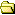All Forums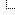Origin Forum for Programming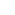Forum for Automation Server/COM and LabVIEW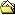matlab loading data from origin workbooks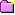New Topic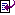Reply to Topic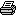Printer Friendly
Author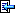Topic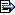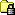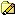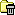Poland
36 Posts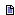Posted - 07/20/2018 :  06:31:49 AM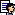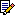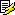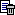Origin Ver. 2018 and Service Release 1 b9.5.1.195 (Select Help-->About Origin): Operating System: Win10Hello,I'm not an experienced user of Origin COM Automation Server, but I used to succesfully use a script based on ImportOrigin downloaded from matlab fileexchange site (https://www.mathworks.com/matlabcentral/fileexchange/43116-importorigin)```tempdata = struct('name',[],'xy',[]); tempdata(1) = []; originObj = actxserver('Origin.ApplicationSI'); workbooksHandle = invoke(originObj,'WorksheetPages'); nbooks = get(workbooksHandle,'Count') % Number of workbooks in project for b = 0:nbooks-1 % Loop all workbooks %workbookHandle = get(workbooksHandle,'Parent',b) workbookHandle = get(workbooksHandle,'Item',b); % Handle to workbook b workbookName = get(workbookHandle,'Name'); % Short name of workbook b workbookLongName = get(workbookHandle,'LongName') % Long name of workbook b % Identify worksheets worksheetsHandle = get(workbookHandle,'layers'); % Handle to worksheets of workbook b nsheets = get(worksheetsHandle,'Count'); % Number of sheets in workbook b for s = 0:nsheets-1 % Loop all worksheets worksheetHandle = get(worksheetsHandle,'Item',s); % Handle to sheet s worksheetName = get(worksheetHandle,'Name'); % Short name of sheet s worksheetLongName = get(worksheetHandle,'LongName'); % Long name of sheet s worksheetData = invoke(originObj,'GetWorksheet',sprintf('[%s]%s',workbookName,worksheetName)); % Get worksheet data fh = @(x) all(isnan(x(:))); worksheetData(cellfun(fh, worksheetData)) = {[]}; % Remove all NaN from cell array fh = @(x) all(ischar(x(:))); worksheetData(cellfun(fh, worksheetData)) = {[]}; % Remove all strings from cell array if ~iscell(worksheetData) || isempty(worksheetData) % If there is no worksheet data found, continue to next continue end % Identify columns columnsHandle = get(worksheetHandle,'Columns'); % Handle to columns of worksheet s ncolumns = get(columnsHandle,'Count'); % Number of columns in sheet s x = []; y = []; for c = 0:ncolumns-1 % Loop all columns columnHandle = get(columnsHandle,'Item',c); % Handle to column c columnName = get(columnHandle,'Name'); % Name of column c columnType = get(columnHandle,'Type'); % Column type: 0 (Y), 3 (X) or 5 (Z) columnLongName = get(columnHandle,'LongName'); % Long name of column c columnsUnits = get(columnHandle,'Units'); % Units specified in column c (not actually used here) columnComment = get(columnHandle,'Comments'); % Comments specified in column c (not actually used here) % Store column data if columnType==3 % If x-vector x = [worksheetData{:,c+1}]'; x(isnan(x)) = []; % Remove NaN elseif columnType==0 % If y-vector y = [worksheetData{:,c+1}]'; y(isnan(y)) = []; % Remove NaN % Check x,y data if ~isnumeric(x) || ~isnumeric(y) || ismember(1,isnan(x)) || ismember(1,isnan(y)) || isempty(x) || isempty(y) % || length(x)<5 || length(y)<5 % If x and y are not actual data, skip to next continue end % Put (x,y) pair into tempdata structure tempdata(end+1).name = sprintf('Book[%s]: Sheet[%s]: Col(%s): Name(%s)',workbookLongName,worksheetName,columnName,columnLongName); if length(x)>length(y) tempdata(end).xy = [x(1:length(y)) y]; % Cut x elseif length(x)

366 PostsPosted - 07/20/2018 :  4:41:09 PMHi,Couple of things need to be changed in your script:1. change iterator index b, c to int32(b), int32(c), etc. (Line 9, 18, 38)2. Column type returns strings, need to change line 46, 49. Please try the following script:``` tempdata = struct('name',[],'xy',[]); tempdata(1) = []; originObj = actxserver('Origin.ApplicationSI'); workbooksHandle = invoke(originObj,'WorksheetPages'); nbooks = get(workbooksHandle,'Count') % Number of workbooks in project for b = 0:nbooks-1 % Loop all workbooks %workbookHandle = get(workbooksHandle,'Parent',b) workbookHandle = get(workbooksHandle,'Item',int32(b)); % Handle to workbook b workbookName = get(workbookHandle,'Name'); % Short name of workbook b workbookLongName = get(workbookHandle,'LongName') % Long name of workbook b % Identify worksheets worksheetsHandle = get(workbookHandle,'layers'); % Handle to worksheets of workbook b nsheets = get(worksheetsHandle,'Count'); % Number of sheets in workbook b for s = 0:nsheets-1 % Loop all worksheets worksheetHandle = get(worksheetsHandle,'Item',int32(s)); % Handle to sheet s worksheetName = get(worksheetHandle,'Name'); % Short name of sheet s worksheetLongName = get(worksheetHandle,'LongName'); % Long name of sheet s worksheetData = invoke(originObj,'GetWorksheet',sprintf('[%s]%s',workbookName,worksheetName)); % Get worksheet data fh = @(x) all(isnan(x(:))); worksheetData(cellfun(fh, worksheetData)) = {[]}; % Remove all NaN from cell array fh = @(x) all(ischar(x(:))); worksheetData(cellfun(fh, worksheetData)) = {[]}; % Remove all strings from cell array if ~iscell(worksheetData) || isempty(worksheetData) % If there is no worksheet data found, continue to next continue end % Identify columns columnsHandle = get(worksheetHandle,'Columns'); % Handle to columns of worksheet s ncolumns = get(columnsHandle,'Count'); % Number of columns in sheet s x = []; y = []; for c = 0:ncolumns-1 % Loop all columns columnHandle = get(columnsHandle,'Item',int32(c)); % Handle to column c columnName = get(columnHandle,'Name'); % Name of column c columnType = get(columnHandle,'Type'); % Column type: 0 (Y), 3 (X) or 5 (Z) columnLongName = get(columnHandle,'LongName'); % Long name of column c columnsUnits = get(columnHandle,'Units'); % Units specified in column c (not actually used here) columnComment = get(columnHandle,'Comments'); % Comments specified in column c (not actually used here) % Store column data if columnType=='COLTYPE_X' % If x-vector x = [worksheetData{:,c+1}]'; x(isnan(x)) = []; % Remove NaN elseif columnType=='COLTYPE_Y' % If y-vector y = [worksheetData{:,c+1}]'; y(isnan(y)) = []; % Remove NaN % Check x,y data if ~isnumeric(x) || ~isnumeric(y) || ismember(1,isnan(x)) || ismember(1,isnan(y)) || isempty(x) || isempty(y) % || length(x)<5 || length(y)<5 % If x and y are not actual data, skip to next continue end % Put (x,y) pair into tempdata structure tempdata(end+1).name = sprintf('Book[%s]: Sheet[%s]: Col(%s): Name(%s)',workbookLongName,worksheetName,columnName,columnLongName); if length(x)>length(y) tempdata(end).xy = [x(1:length(y)) y]; % Cut x elseif length(x)

Poland
36 PostsPosted - 07/23/2018 :  08:31:42 AMYes, now it works! Thank you very much.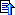TopicNew TopicReply to TopicPrinter Friendly Jump To: Select Forum Origin Forum       Origin Viewer Forum       Origin Forum Origin Forum for Programming       Forum for Python       LabTalk Forum       Forum for Origin C       Forum for Automation Server/COM and LabVIEW Origin中文论坛       Origin 中文论坛 (Chinese Origin Forum) Japanese Origin Forum       Origin日本語フォーラム (Japanese Origin Forum) Origin on Linux       The Origin on Linux Forum Private Forums       Distributor Forum  -------------------- Home Active Topics Frequently Asked Questions Member Information Search Page
 The Origin Forum © 2020 Originlab Corporation